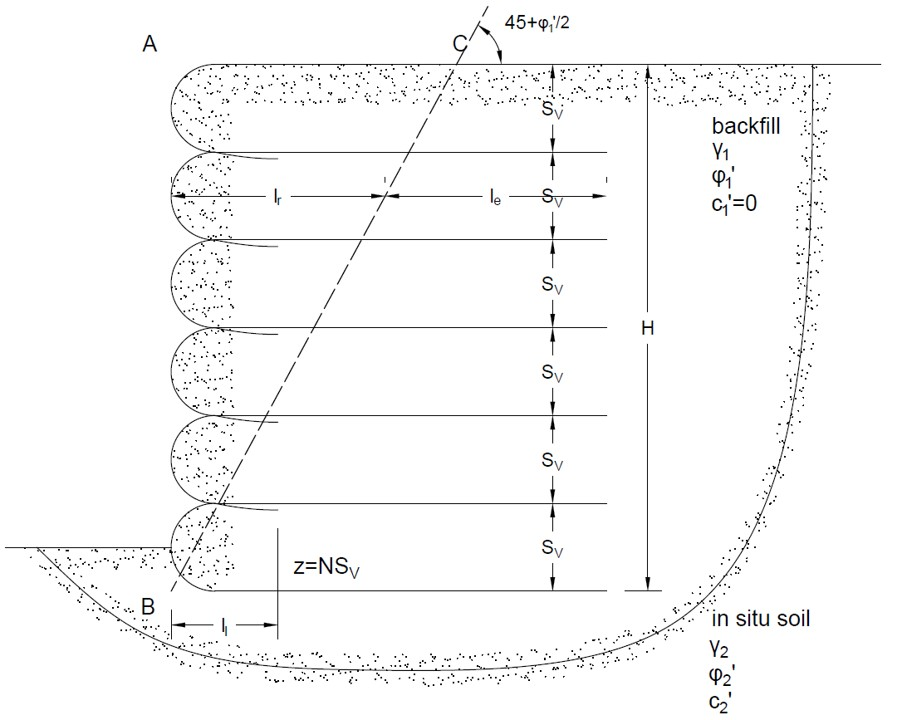# Retaining Wall Tool (Geotextile)## Geometry of retaining wall with geotextile reinforcement## Tips

• This tool is for analysis of retaining walls with geotextile reinforcement, as shown in the figure above.
• Two homogenous soil layers are considered in the analysis.
• The Rankine earth pressure theory is used.
• No surcharge is considered in this case.
• For the backfill, the cohesion c1 is assumed to be zero.
• The height of retaining wall H is suggested to be an integral multiple of SV vertical spacing of layers.
• The friction angle at geotextile-soil interface is assumed as 2/3φ′1.
• All the input parameters should be positive.
• For "nan", "0" or "inf" displayed in Results, please check your input parameters.

γ1 (kN/m3)
φ′1 (°)
γ2 (kN/m3)
φ′2 (°)
c2 (kN/m2)
H (m)
Tult (kN/m)
FSB
FSP
RFid
RFcr
RFcbd

## Output Results

• Allowable tensile strength
Tall (kN/m) =
• Vetcial spacing of geotextile layers
SV (m) =
• Minimum geotextile layer length
L (m) =
• Minimum lap length
ll (m) =
• Safety factor of overturning
FSO =
• Safety factor of sliding
FSS =
• Safety factor of bearing capacity
FSBC =